Finally, a Math Education Tool that Supports Research-Based Learning Theory!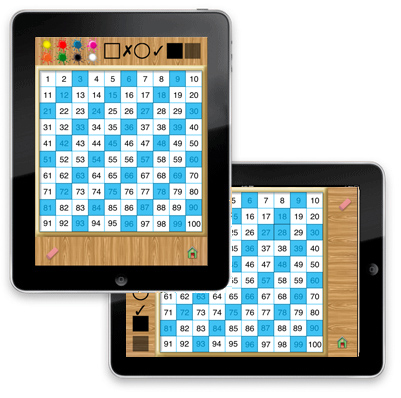Hands-On Math: Interactive Hundreds Chart creates a virtual math playground where students explore, investigate and discover mathematical concepts. Students use colored markers on the Interactive Hundreds Chart to represent patterns and number sequences. Using Hands-On Math: Interactive Hundreds Chart students can develop the following important mathematical concepts: Skip-Counting Number Patterns Multiplication and Division Facts Prime and Composite Numbers Least Common Multiple Divisibility of Numbers and more. We recommend that you open the Instructor's Guide PDF from your iPad in an new page and save the document in your iBooks app. Link to PiPad YouTube video by Professor Lamb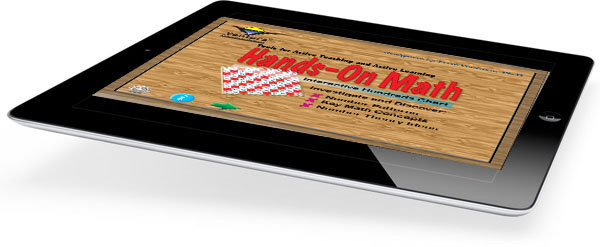Features: Open-ended Interactive Hundreds Chart Playground. Supports Discovery and Directed Teaching Strategies . Problem Solving Activities which Can Be Solved Using Virtual Manipulatives. The Interactive Hundreds Chart helps to Build a Fundamental Knowledge of Number Theory. On/Off Options and Controls for Various Features. \$2.99
 Interactive Hundreds Chart Playground Colorful markers are used to indicate patterns and sequences. The playground offers an ample supply of markers to be placed, moved, arranged, and grouped in order to represent mathematical ideas. Topics: Skip-Counting Patterns and Multiples Even and Odd Prime and Composite Least Common Multiple LCM Equivalency Addition, Subtraction, Multiplication and Division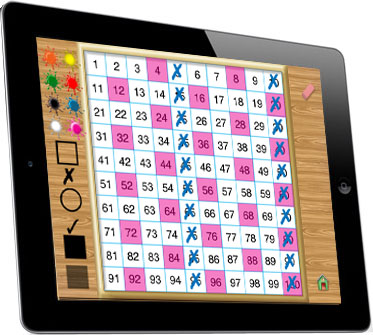Interactive Hundreds Chart Finding the Least Common Multiple Choose a color and marker. Build a pattern (for example, 4, 8, 12, 16...) Choose a different color and marker. Build a second pattern (for example, 5, 10, 15, 20...) Locate the first number with two marks. This is the Least Common Multiple (LCM) Quick-Check Problem Sets Activities at a variety of skill levels are also provided. Correctly marking the answers on the Hundreds Chart produces a pattern or design, which can easily be checked by the teacher with just a glance at the student's iPad. Perfect for Small Group instruction Environmentally Friendly Learning - (no need to photocopy Hundreds Charts for each student) A Student-Centered Approach to Learning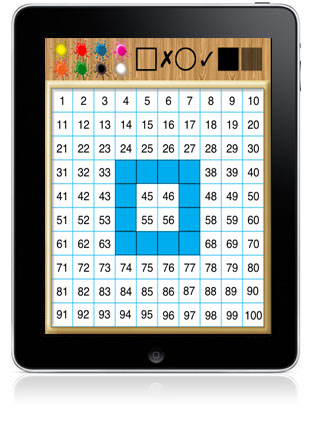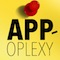App-oplexy Review Read how a teacher is using Hands-On Math Hundreds Chart to create individualized instructional programs that meet Common Core Standards and IEP goals for her students.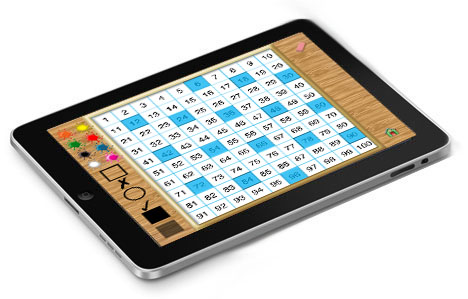Hands-On Math: Interactive Hundreds Chart is now available at the Apple iTunes Store.

 Use our Interactive Hundreds Chart to address these Common Core Standards Grade Standard 1 Count to 100. In this range, read and write numerals and represent a number of objects with a written numeral. (Standard extends counting to 120.) Use our Interactive Hundreds Chart to address this standard by setting the speech function to ON. As students tap numbers to place markers they will hear the numbers spoken. Practice counting by 2, 3, or 4, and so on. 2 Add and Subtract within 20. Assist students in mastering this standard by assigning addition and subtraction problems. Place markers on the Hundreds Chart to show the answers to these problems. Let the answers form a pattern or picture. Work with equal groups of objects to gain foundations for multiplication. Practice the multiples of the numbers from 2 to 10 by marking patternso the Hundreds Chart 3 Represent and solve problems involving multiplication and division. Pick a composite number from 1 to 100. Ask students to mark a pattern showing the factors of the number. Interpret products of whole numbers, e.g., interpret 5 × 7 as the total number of objects in 5 groups of 7 objects each. Mark a pattern showing the multiples of the two factors, for example, 5 and 7. Notice that 35 is marked in both patterns. (i.e, 5, 10, 15, 20, 25, 30, 35, 40... and ( 7, 14, 21, 28, 35...) 4 Interpret a multiplication equation as a comparison, e.g., interpret 35 = 5 × 7 as a statement that 35 is 5 times as many as 7 and 7 times as many as 5. Represent verbal statements of multiplicative comparisons as multiplication equations. Find all factor pairs for a whole number in the range 1–100. Recognize that a whole number is a multiple of each of its factors. Determine whether a given whole number in the range 1–100 is a multiple of a given one-digit number. Determine whether a given whole number in the range 1–100 is prime or composite. Use the Hundreds Chart to demonstrate the Sieve of Eratosthenes. Generate a number or shape pattern that follows a given rule. Identify apparent features of the pattern that were not explicit in the rule itself. 5 Add and subtract fractions with unlike denominators (including mixed numbers) by replacing given fractions with equivalent fractions in such a way as to produce an equivalent sum or difference of fractions with like denominators. Use the Hundreds Chart to overlay two patterns, for example, 5 and 7. Notice that 35 is the first number that occurs in both patterns. 35 is the LCM of 5 and 7.